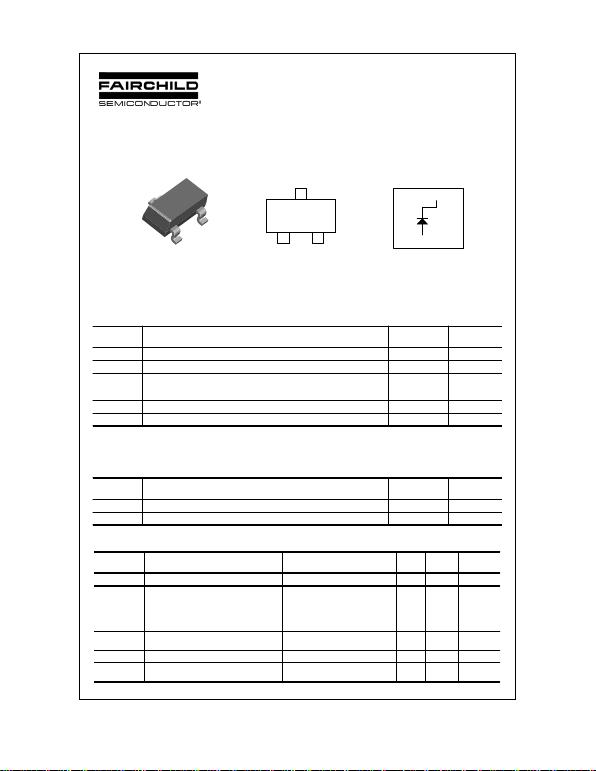BAS29
BAS29, Rev. A
BAS29
Small Signal Diode
*These ratings are limiting values above which the serviceability of any semiconductor device may be impaired.
NOTES:
1) These ratings are based on a maximum junction temperature of 150 degrees C.
2) These are steady state limits. The factory should be consulted on applications involving pulsed or low duty cycle operations.
2002 Fairchild Semiconductor Corporation
3
1
2
SOT-23
12
3
L20
Absolute Maximum Ratings* TA = 25°C unless otherwise noted
Symbol
Parameter
Value
Units
VRRM Maximum Repetitive Reverse Voltage 120 V
IF(AV) Average Rectified Forward Current 200 mA
IFSM Non-repetitive Peak Forward Surge Current
Pulse Width = 1.0 second
Pulse Width = 1.0 microsecond
1.0
2.0
A
A
Tstg Storage Temperature Range -55 to +150 °C
TJ Operating Junction Temperature 150 °C
Thermal Characteristics
Electrical Characteristics TA = 25°C unless otherwise noted
Symbol
Parameter
Value
Units
PD Power Dissipation 350 mW
RθJA Thermal Resistance, Junction to Ambient 357 °C/W
Symbol
Parameter Test Conditions Min
Max
Units
VR Breakdown Voltage IR = 1.0 mA 120 V
VF* Forward Voltage IF = 10 mA
IF = 50 mA
IF = 100 mA
IF = 200 mA
IF = 400 mA
0.75
0.84
0.90
1.00
1.25
V
V
V
V
V
IR* Reverse Current VR = 90 V
VR = 90 V, TA = 150°C 100
100 nA
µA
CT Total Capacitance VR = 0, f = 1.0 MHz 2.0 pF
trr Reverse Recovery Time IF = IR = 30 mA, IRR = 3.0 mA,
RL = 100 50 ns
*Pulse test : Pulse width=300us, Duty Cycle=2%
Connection Diagram
3
12NC